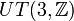# Central product of UT(3,Z) and Z identifying center with 2Z

View a complete list of particular groups (this is a very huge list!)[SHOW MORE]

## Definition

This group can be defined in the following equivalent ways:

1. It is the central product of unitriangular matrix group:UT(3,Z) and the group of integers$\mathbb{Z}$ where the subgroup$2\mathbb{Z}$ of$\mathbb{Z}$ is identified with the center of$UT(3,\mathbb{Z})$.
2. It is the following group of matrices under multiplication:$\left\{ \begin{pmatrix} 1 & a & b \\ 0 & 1 & c \\ 0 & 0 & 1 \\\end{pmatrix} \mid a,c \in \mathbb{Z}, 2b \in \mathbb{Z} \right \}$

This group is almost like unitriangular matrix group:UT(3,Z). In fact,$UT(3,\mathbb{Z})$ occurs as a subgroup of index two inside it. However, unlike$UT(3,\mathbb{Z})$, it is a CS-Baer Lie group, and hence can participate in the CS-Baer correspondence.

## Arithmetic functions

Function Value Similar groups Explanation
nilpotency class 2
derived length 2
Frattini length 2
Hirsch length 3